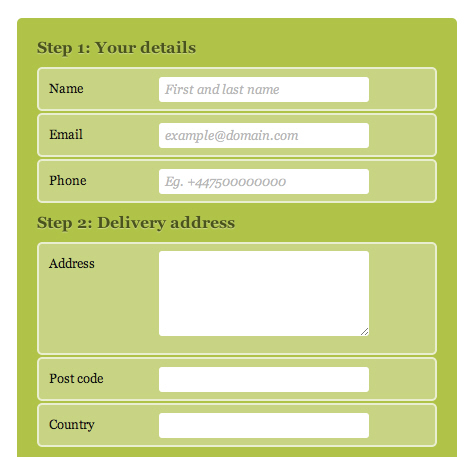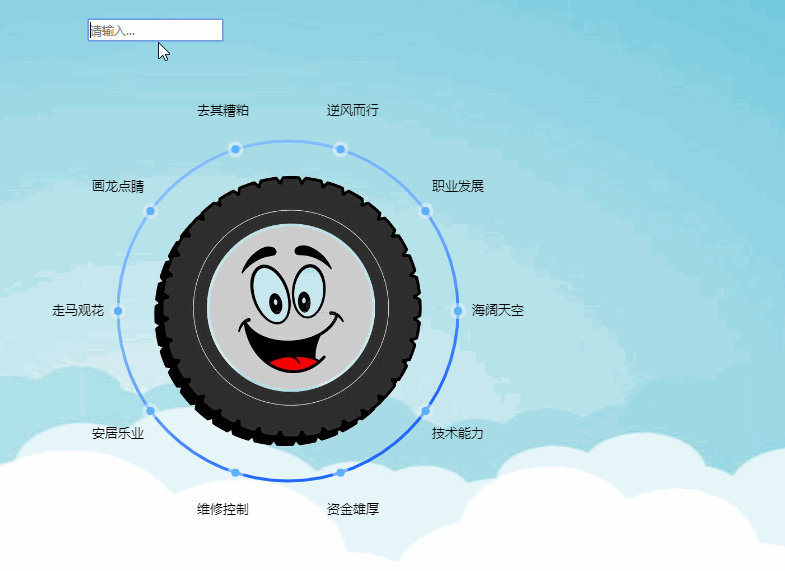# 使用html5 canvas绘制圆环动效_html5教程技巧​ ​​​ ​​

html部分代码如下：

<canvas style="margin-left: 50px;padding-top: 20px; display:block;" id="canvas" > 您的浏览器当前版本不支持canvas</canvas>

1、绘制大圆环。

canvas.width = 500canvas.height = 500//计算画布中心位置的半径let r = 500 / 2// 界面初始化的时候将画布的原点移动至画布中心ctx.translate(r,r) //将画笔移动到圆形

// 画布初始化let canvas = document.getElementById('canvas')let ctx= canvas.getContext('2d')let ratio = getPixelRato(ctx)canvas.width = 500canvas.height = 500//计算画布中心位置的半径let r = 500 / 2// 界面初始化的时候将画布的原点移动至画布中心ctx.translate(r,r) //将画笔移动到圆形ctx.lineWidth = 3; //设置画笔的线宽ctx.beginPath(); //画笔开始// 绘制圆环边缘渐变边缘颜色var arcColor = ctx.createLinearGradient(-170, -170, 0, 170)arcColor.addColorStop(0, '#8ec1ff')arcColor.addColorStop(0.2, '#83beff')arcColor.addColorStop(0.5, '#75b1ff')arcColor.addColorStop(0.7,'#5998ff')arcColor.addColorStop(1, '#2065ff')ctx.strokeStyle= arcColor;//设置画笔的颜色ctx.arc(0,0,r - 80,0,2*Math.PI,false) //绘制圆形，坐标0,0,半径250-80，整圆（0-360度），false表示顺时针ctx.closePath()ctx.stroke() //绘图

​绘制结果如下2、绘制圆环中部背景图片（当前画布原点为画布中心）

drawImage(image, dx, dy, dWidth, dHeight)

image：Canvas图片资源，如<img>图片，SVG图像，Canvas元素本身等。

dx、dy：在Canvas画布上规划一片区域用来放置图片，dx就是这片区域的左上角横、纵坐标。

dWidth、dHeight：在Canvas画布上规划一片区域用来放置图片，这片区域的宽度、高度。

let image = new Image()image.src = 'image/quan.png'image.onload = () => { // 原点移动至中心 ctx.drawImage(image,-140,-140,280,280)}3、绘制圆环上的文字，小圆点（当前画布原点为画布中心）

3.1 小圆点均匀的显示在大圆环上

3.2 文字散落在小圆点外方一点点

1、笔者使用一个数组来存储当前的词语

let textArr = ['海阔天空','技术能力','资金雄厚','维修控制','安居乐业','走马观花','画龙点睛','去其糟粕','逆风而行','职业发展']

2、因为小圆点的个数以及词语的个数是一样的，它们两个的个数也就是上方数组textArr的length

3、一个整圆的弧度是2π，要让小圆点们均分圆环，笔者首先计算出每个小圆点所在点的弧度

for(let i = 0;i<lengths;i++){ // 计算弧度 let rad = 2*Math.PI/lengths*i}

4、根据三角函数可以计算出当前小圆点在画布上的坐标（x,y）（当前画布原点为画布中心）// 计算小圆心坐标let x = (r - 40)*Math.cos(rad)let y = (r - 40)*Math.sin(rad)

// 计算文字的坐标 let x = (r - 80)*Math.cos(rad) let y = (r - 80)*Math.sin(rad)

// 绘制文字ctx.font = '13px Arial'ctx.textAlign = 'center'ctx.textBaseline = 'middle'ctx.fillStyle="#000000"let lengths = textArr.lengthtextArr.forEach(function(text,i){ //弧度 let rad = 2*Math.PI/lengths*i // 计算小圆心坐标 let x = (r - 40)*Math.cos(rad) let y = (r - 40)*Math.sin(rad) ctx.fillText(text,x+0.5,y+0.5)});// 绘制小圆点for(let i = 0;i<lengths;i++){ // // let rad = 2*Math.PI/lengths*i let x = (r - 80)*Math.cos(rad) let y = (r - 80)*Math.sin(rad)// // 绘制边缘灰色半透明小圆点 ctx.beginPath() ctx.fillStyle = 'rgba(226,235,250,0.8)' ctx.arc(x,y,8,0,2*Math.PI,false) ctx.closePath() ctx.fill() // 绘制蓝色小圆点 ctx.beginPath() ctx.fillStyle = '#208fe5' ctx.arc(x,y,4,0,2*Math.PI,false) ctx.closePath() ctx.fill() }4、绘制每个小圆点外面的三角形（当前画布原点为画布中心）

4.1 因为要绘制出三角形的形状，绘制三角形的思路就是，以当前小圆点的圆心为起点向两侧画条线，然后使用ctx.fill()封闭图形，并使用渐变色填充内部。

//画笔开始 ctx.beginPath() ctx.moveTo(x,y) ctx.lineTo(x-35,y+70) ctx.lineTo(x+35,y+70) ctx.closePath()

ctx.fillStyle= '#e3211c' ctx.fillText(textArr[i],x,y+75)

for(let i = 0;i<lengths;i++){ // // let rad = 2*Math.PI/lengths*i let x = (r - 80)*Math.cos(rad) let y = (r - 80)*Math.sin(rad) // // 画s三角形 // // ctx.rotate( -Math.PI / 4) ctx.beginPath() //画笔开始 ctx.moveTo(x,y) ctx.lineTo(x-35,y+70) ctx.lineTo(x+35,y+70) ctx.closePath() // // 设置 颜色 渐变--->从中心向两边添加颜色 var sColor = ctx.createLinearGradient (x,y,x+18,y+50) sColor.addColorStop(0,'rgba(106,128,243,0.5)') sColor.addColorStop(0.6,'rgba(83,183,243,0.5)') sColor.addColorStop(0.7,'rgba(129,200,224,0.5)') sColor.addColorStop(0.8,'rgba(130,219,251,0.5)') sColor.addColorStop(1,'rgba(195,228,223,0.5)') ctx.fillStyle= sColor ctx.fill() ctx.fillStyle= '#e3211c' ctx.fillText(textArr[i],x,y+75)}4.2 需求是每个三角形的方向是向外散发，而现在三角形的方向都是朝下方，所以现在需要使用canvas的旋转方法。

ctx.save() ctx.translate(x,y) // 旋转角度以每个小圆点为中心 ctx.rotate( rad - Math.PI/2 ) // 因为一开始小圆点 ctx.translate(-x, -y) . 省略画三角形和文字的代码 . . ctx.restore()

restore()，依次从堆栈的上方弹出存储的Canvas状态，如果没有任何存储的Canvas状态，则执行此方法没有任何变化。

for(let i = 0;i<lengths;i++){ // // let rad = 2*Math.PI/lengths*i let x = (r - 80)*Math.cos(rad) let y = (r - 80)*Math.sin(rad) // 画s三角形 ctx.save() // 旋转角度以每个小圆点为中心 因为一开始小圆点 ctx.translate(x,y) ctx.rotate( rad - Math.PI/2 ) ctx.translate(-x, -y) // 画笔开始 ctx.beginPath() ctx.moveTo(x,y) ctx.lineTo(x-35,y+70) ctx.lineTo(x+35,y+70) ctx.closePath() //设置 颜色 渐变--->从中心向两边添加颜色 var sColor = ctx.createLinearGradient (x,y,x+18,y+50) sColor.addColorStop(0,'rgba(106,128,243,0.5)') sColor.addColorStop(0.6,'rgba(83,183,243,0.5)') sColor.addColorStop(0.7,'rgba(129,200,224,0.5)') sColor.addColorStop(0.8,'rgba(130,219,251,0.5)') sColor.addColorStop(1,'rgba(195,228,223,0.5)') ctx.fillStyle= sColor ctx.fill() ctx.fillStyle= '#e3211c' ctx.fillText(textArr[i],x,y+75) ctx.restore()}function rotateContext(ctx, x, y, degree) { // 旋转文字 ctx.translate(x, y) // ctx.rotate(degree * Math.PI / 180) ctx.rotate(degree) ctx.translate(-x, -y) }

if (rad > Math.PI) { // 因为文字需要显示在三角形的边缘，所以文字应该随着三角形旋转，才能一直维持在 // 三角形的边缘，由于旋转后当弧度大于π的值都会出现文字倒转问题，于是将文字进行旋转翻转 ctx.save() ctx.beginPath() // 旋转文字 rotateContext(ctx, x, y+75, Math.PI) ctx.font = '13px Arial' ctx.textAlign = 'center' ctx.fillStyle = "#ff2238" ctx.fillText(textArr[i], x, y+ 75) ctx.restore()} else { ctx.fillStyle = '#ff2238' ctx.fillText(textArr[i], x, y + 75)}5、下面就是实现，鼠标在小圆点上方，让边缘的三角形和三角形边缘文字显示，而圆环边的文字不显示

1、给画布绑定鼠标进入事件

2、判断当前鼠标所在画布位置的坐标是否等于某个小圆点的附近的坐标，如果等于就显示对应小圆点的三角形。

5.1给canvas画布绑定mousemove事件：鼠标在上方事件

5.2 计算鼠标当前在画布上的坐标

function clickEvent() { // 鼠标所在位置坐标 let x = event.clientX - canvas.getBoundingClientRect().left let y = event.clientY - canvas.getBoundingClientRect().top drawOne(x,y)}

5.3，因为上方计算出来的鼠标在画布上的坐标是以画布的左上角为原点计算的坐标，但是当前画布的原点早已移动到画布中心（250,250）处，所以当用来判断是否是点击某个小圆点的时候需要横纵坐标都减去250，才能与当前画布的小圆点坐标进行比哦对，笔者在判断的时候，发现 一个问题，不知道为啥笔者的y方向的差量是260而不是250，所以笔者y方向上都减去了260。

let XX = Cx - 250let YY = Cy- 260let leftX = x - 15 let rightX = x + 15let topY = y - 15let bottomY = y + 15if (XX >= leftX && XX <= rightX && YY <= bottomY && YY >= topY ) {//就是它被点了。。。。。。//这中间写绘制的代码}

6，界面上定义一个Input，给input绑定change事件。

html代码：

<input type="text" id="inpt" style="margin-left: 100px;margin-top: 50px" placeholder="请输入...">

js代码：

let inpt = document.getElementById('inpt') inpt.addEventListener('change', function () { if (inpt.value !== '') { textArr.push(inpt.value) drawAll(2) //此方法是绘制的方法，文章后续给源代码 }})

7，出现了一个问题，当每次点击界面，重绘界面的时候都会出现一闪一闪的状况clearRect()在Canvas动画绘制中非常常用，不断清除画布内容再绘制，形成动画效果。

clearRect()可以把Canvas元素画布中的某一块矩形区域变成透明的。

context.clearRect(x, y, width, height);

x、y：矩形左上角x、y坐标。

width、heigh：被清除的矩形区域的宽度、高度。

drawImage(image, dx, dy, dWidth, dHeight)

html代码：

<canvas width="280" height="280" style="margin-left: 50px;padding-top: 20px; display:none;" id="canvas2"> </canvas>

js代码：

// 利用缓存来解决重绘图片闪动问题var tempCanvas = document.getElementById('canvas2')const tempCtx = tempCanvas.getContext('2d')tempCanvas.width = 280; tempCanvas.height = 280let image = new Image()image.src = 'image/quan.png'image.onload = () => { // 原点移动至中心 tempCtx.drawImage(image,0,0,280,280)}

// 将缓存的canvas直接绘制到界面（缓存了中间轮胎界面） ctx.drawImage(tempCanvas,-140,-140)https://github.com/Linefate/Dynamic-effect-of-canvas-ring.git

• 本文相关：
• html5实现的3d立体圆环旋转动画效果源码
• 关于html5 canvas 绘图动画的问题,我要实现把一张图片绘制在canvas中,然后使其动起来,代码如下,
• 使用HTML5 Canvas 画出以下图形！
• 如何用HTML5 Canvas制作子画面动画
• 使用HTML5　Canvas如何绘制下面的脸？
• 请教关于HTml5的问题，基于canvas 的绘图怎么样进行各种绘图操作?
• 如何用js获得html5中用canvas画出来的对象？急
• HTML5用canvas怎么画出如下效果呢？
• 如何使用HTML5 Canvas动态的绘制拓扑图
• 如何使用HTML5 Canvas动态的绘制拓扑图
• 如何使用HTML5 Canvas动态的绘制拓扑图
• 免责声明 - 关于我们 - 联系我们 - 广告联系 - 友情链接 - 帮助中心 - 频道导航